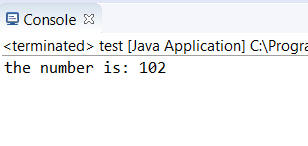# How to Convert a String to Int in Java: Methods and Examples

Published on Oct 01,2019 52 ViewsThere are many methods to convert a string to integer in Java. The method most commonly used is the pareseInt() method. There are multiple methods to convert string to an integer. In this article, we will have a look at all the possible methods to convert a String to int in Java

The three main methods are:

## parseInt() method to convert a String to Int in Java

In this method, the string is converted to a primitive int.

Syntax: `int dtypename = Integer.parseInt(string);`

Example: `int res = Integer.parseInt(str);`

Consider the code:

```public class test {
public static void main(String args[])
{
String str = "102";
int res = Integer.parseInt(str);
System.out.println("the number is: "+ res);
}
}```

OUTPUT:

##EXPLANATION:

In the above code, we have a string variable “102”. We declare another variable res. This is of integer type. So the string variable is converted to an integer by using the parseInt() method.

## valueOf() method: String to Int in Java

In this method, the string is converted to an Integer object.

Syntax: `Integer dtypename = Integer.valueOf(string);`

Example: Integer res = Integer.valueOf(str);

Consider the code:

```public class test {
public static void main(String args[])
{
String str = "102";
int res = Integer.parseInt(str);
System.out.println("the number is: "+ res);
}
}```

OUTPUT:

##EXPLANATION:

In the above code, we have a string variable “122”. We declare another variable result as res. This is of integer type. So the string variable is converted to an integer by using the valueOf() method.

NOTE: parseInt() returns a primitive int whereas valueOf() returns a new Integer() object.

## NumberFormatException Method

A NumberFormatException is thrown when the string is not parsable.

Consider the code:

```public class test {
public static void main(String args[])
{
String str = "102";
int res = Integer.parseInt(str);
System.out.println("the number is: "+ res);
}
}```

OUTPUT:

##EXPLANATION:

An exception is generated when the string is not parsable.

With this, we come to an end of this String to Int in Java article. I hope you got an idea of all the three methods of Converting a String to int in Java.

Check out the Java Training by Edureka, a trusted online learning company with a network of more than 250,000 satisfied learners spread across the globe. We are here to help you with every step on your journey, for becoming a besides this java interview questions, we come up with a curriculum which is designed for students and professionals who want to be a Java Developer.REGISTER FOR FREE WEBINARThank you for registering Join Edureka Meetup community for 100+ Free Webinars each month